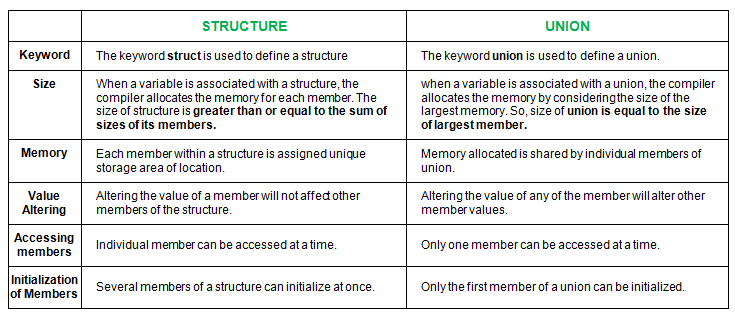Open in App
Not now

# Difference between Structure and Union in C

• Difficulty Level : Easy
• Last Updated : 10 Nov, 2022

Structures in C is a user-defined data type available in C that allows to combining of data items of different kinds. Structures are used to represent a record.

Defining a structure: To define a structure, you must use the struct statement. The struct statement defines a new data type, with more than or equal to one member. The format of the struct statement is as follows:

```   struct [structure name]
{
member definition;
member definition;
...
member definition;
};

(OR)

struct [structure name]
{
member definition;
member definition;
...
member definition;
}structure variable declaration;```

Union in C is a special data type available in C that allows storing different data types in the same memory location. You can define a union with many members, but only one member can contain a value at any given time. Unions provide an efficient way of using the same memory location for multiple purposes.

Defining a Union: To define a union, you must use the union statement in the same way as you did while defining a structure. The union statement defines a new data type with more than one member for your program. The format of the union statement is as follows:

```    union [union name]
{
member definition;
member definition;
...
member definition;
};

(OR)

union [union name]
{
member definition;
member definition;
...
member definition;
}union variable declaration;```

### Similarities between Structure and Union

1. Both are user-defined data types used to store data of different types as a single unit.
2. Their members can be objects of any type, including other structures and unions or arrays. A member can also consist of a bit field.
3. Both structures and unions support only assignment = and sizeof operators. The two structures or unions in the assignment must have the same members and member types.
4. A structure or a union can be passed by value to functions and returned by value by functions. The argument must have the same type as the function parameter. A structure or union is passed by value just like a scalar variable as a corresponding parameter.
5. ‘.’ operator or selection operator, which has one of the highest precedences, is used for accessing member variables inside both the user-defined datatypes.

Differences between Structure and Union are as shown below in tabular format as shown below as follows:## C

 `// C program to illustrate differences``// between structure and Union` `#include ``#include `` ` `// declaring structure``struct` `struct_example``{``    ``int` `integer;``    ``float` `decimal;``    ``char` `name;``};`` ` `// declaring union`` ` `union` `union_example``{``    ``int` `integer;``    ``float` `decimal;``    ``char` `name;``};`` ` `void` `main()``{``    ``// creating variable for structure``    ``// and initializing values difference``    ``// six``    ``struct` `struct_example s={18,38,``"geeksforgeeks"``};`` ` `    ``// creating variable for union``    ``// and initializing values``    ``union` `union_example u={18,38,``"geeksforgeeks"``};`` ` `         ` `    ``printf``(``"structure data:\n integer: %d\n"``                ``"decimal: %.2f\n name: %s\n"``,``                ``s.integer, s.decimal, s.name);``    ``printf``(``"\nunion data:\n integer: %d\n"``                 ``"decimal: %.2f\n name: %s\n"``,``                ``u.integer, u.decimal, u.name);`` ` ` ` `    ``// difference two and three``    ``printf``(``"\nsizeof structure : %d\n"``, ``sizeof``(s));``    ``printf``(``"sizeof union : %d\n"``, ``sizeof``(u));``     ` `    ``// difference five``    ``printf``(``"\n Accessing all members at a time:"``);``    ``s.integer = 183;``    ``s.decimal = 90;``    ``strcpy``(s.name, ``"geeksforgeeks"``);``     ` `    ``printf``(``"structure data:\n integer: %d\n "``                ``"decimal: %.2f\n name: %s\n"``,``            ``s.integer, s.decimal, s.name);``     ` `    ``u.integer = 183;``    ``u.decimal = 90;``    ``strcpy``(u.name, ``"geeksforgeeks"``);``     ` `    ``printf``(``"\nunion data:\n integer: %d\n "``                ``"decimal: %.2f\n name: %s\n"``,``            ``u.integer, u.decimal, u.name);``     ` `    ``printf``(``"\n Accessing one member at time:"``);``     ` `    ``printf``(``"\nstructure data:"``);``    ``s.integer = 240;``    ``printf``(``"\ninteger: %d"``, s.integer);``     ` `    ``s.decimal = 120;``    ``printf``(``"\ndecimal: %f"``, s.decimal);``     ` `    ``strcpy``(s.name, ``"C programming"``);``    ``printf``(``"\nname: %s\n"``, s.name);``     ` `    ``printf``(``"\n union data:"``);``    ``u.integer = 240;``    ``printf``(``"\ninteger: %d"``, u.integer);``     ` `    ``u.decimal = 120;``    ``printf``(``"\ndecimal: %f"``, u.decimal);``     ` `    ``strcpy``(u.name, ``"C programming"``);``    ``printf``(``"\nname: %s\n"``, u.name);``     ` `    ``//difference four``    ``printf``(``"\nAltering a member value:\n"``);``    ``s.integer = 1218;``    ``printf``(``"structure data:\n integer: %d\n "``                ``" decimal: %.2f\n name: %s\n"``,``                ``s.integer, s.decimal, s.name);``     ` `    ``u.integer = 1218;``    ``printf``(``"union data:\n integer: %d\n"``           ``" decimal: %.2f\n name: %s\n"``,``            ``u.integer, u.decimal, u.name);``}`

Output:

```structure data:
integer: 18
decimal: 38.00
name: geeksforgeeks

union data:
integer: 18
decimal: 0.00
name: ?

sizeof structure: 28
sizeof union: 20

Accessing all members at a time: structure data:
integer: 183
decimal: 90.00
name: geeksforgeeks

union data:
integer: 1801807207
decimal: 277322871721159510000000000.00
name: geeksforgeeks

Accessing one member at a time:
structure data:
integer: 240
decimal: 120.000000
name: C programming

union data:
integer: 240
decimal: 120.000000
name: C programming

Altering a member value:
structure data:
integer: 1218
decimal: 120.00
name: C programming
union data:
integer: 1218
decimal: 0.00
name: ?```

Note: structures are better than unions since memory is shared in a union which results in a bit of ambiguity. But technically speaking, unions are better in that they help save a lot of memory, resulting in the overall advantage over structures in the long run.
Quiz on structures and Union

This article is contributed by Harish Kumar. If you like GeeksforGeeks and would like to contribute, you can also write an article using write.geeksforgeeks.org or mail your article to review-team@geeksforgeeks.org. See your article appearing on the GeeksforGeeks main page and help other Geeks.